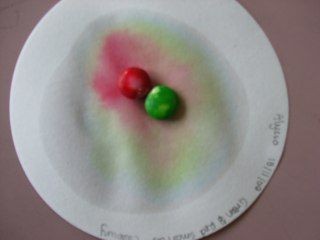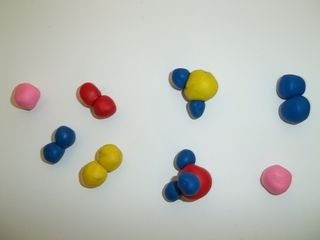Home Practice
For learners and parents For teachers and schools
Textbooks
Full catalogue
Pricing SupportLog in

We think you are located in United States. Is this correct?

# 2.3 Pure substances

## 2.3 Pure substances (ESAZ)

Any material that is not a mixture, is called a pure substance. Pure substances include elements and compounds. It is much more difficult to break down pure substances into their parts, and complex chemical methods are needed to do this.

We can use melting and boiling points and chromatography to test for pure substances. Pure substances have a sharply defined (one temperature) melting or boiling point. Impure substances have a temperature range over which they melt or boil. Chromatography is the process of separating substances into their individual components. If a substance is pure then chromatography will only produce one substance at the end of the process. If a substance is impure then several substances will be seen at the end of the process.

## Recommended practical activity: Smartie chromatography

You will need:

• filter paper (or blotting paper)

• some smarties in different colours

• water

• an eye dropper.

Place a smartie in the centre of a piece of filter paper. Carefully drop a few drops of water onto the smartie, until the smartie is quite wet and there is a ring of water on the filter paper. After some time you should see a coloured ring on the paper around the smartie. This is because the food colouring that is used to make the smartie colourful dissolves in the water and is carried through the paper away from the smartie.

Smartie chromatography### Elements (ESAAA)

An element is a chemical substance that can't be divided or changed into other chemical substances by any ordinary chemical means. The smallest unit of an element is the atom.

Element

An element is a substance that cannot be broken down into other substances through chemical means.

There are $$\text{112}$$ officially named elements and about $$\text{118}$$ known elements. Most of these are natural, but some are man-made. The elements we know are represented in the periodic table, where each element is abbreviated to a chemical symbol. Table 2.2 gives the first $$\text{20}$$ elements and some of the common transition metals.

Recently it was agreed that two more elements would be added to the list of officially named elements. These are elements number $$\text{114}$$ and $$\text{116}$$. The proposed name for element $$\text{114}$$ is flerovium and for element $$\text{116}$$ it is moscovium. This brings the total number of officially named elements to $$\text{114}$$.

 Element name Element symbol Element name Element symbol Hydrogen $$\text{H}$$ Phosphorus $$\text{P}$$ Helium $$\text{He}$$ Sulfur $$\text{S}$$ Lithium $$\text{Li}$$ Chlorine $$\text{Cl}$$ Beryllium $$\text{Be}$$ Argon $$\text{Ar}$$ Boron $$\text{B}$$ Potassium $$\text{K}$$ Carbon $$\text{C}$$ Calcium $$\text{Ca}$$ Nitrogen $$\text{N}$$ Iron $$\text{Fe}$$ Oxygen $$\text{O}$$ Nickel $$\text{Ni}$$ Fluorine $$\text{F}$$ Copper $$\text{Cu}$$ Neon $$\text{Ne}$$ Zinc $$\text{Zn}$$ Sodium $$\text{Na}$$ Silver $$\text{Ag}$$ Magnesium $$\text{Mg}$$ Platinum $$\text{Pt}$$ Aluminium $$\text{Al}$$ Gold $$\text{Au}$$ Silicon $$\text{Si}$$ Mercury $$\text{Hg}$$

Table 2.2: List of the first 20 elements and some common transition metals

### Compounds (ESAAB)

A compound is a chemical substance that forms when two or more different elements combine in a fixed ratio. Water ($$\text{H}_{2}\text{O}$$), for example, is a compound that is made up of two hydrogen atoms for every one oxygen atom. Sodium chloride ($$\text{NaCl}$$) is a compound made up of one sodium atom for every chlorine atom. An important characteristic of a compound is that it has a chemical formula, which describes the ratio in which the atoms of each element in the compound occur.

Compound

A substance made up of two or more different elements that are joined together in a fixed ratio.

Figure 2.4 might help you to understand the difference between the terms element, mixture and compound. Iron ($$\text{Fe}$$) and sulfur ($$\text{S}$$) are two elements. When they are added together, they form a mixture of iron and sulfur. The iron and sulfur are not joined together. However, if the mixture is heated, a new compound is formed, which is called iron sulfide ($$\text{FeS}$$).

Figure 2.4 showed a submicroscopic representation of a mixture. In a submicroscopic representation we use circles to represent different elements. To show a compound, we draw several circles joined together. Mixtures are simply shown as two or more individual elements in the same box. The circles are not joined for a mixture.

We can also use symbols to represent elements, mixtures and compounds. The symbols for the elements are all found on the periodic table. Compounds are shown as two or more element names written right next to each other. Subscripts may be used to show that there is more than one atom of a particular element. (e.g.$$\text{H}_{2}\text{O}$$ or $$\text{NH}_3$$). Mixtures are written as: a mixture of element (or compound) A and element (or compound) B. (e.g. a mixture of $$\text{Fe}$$ and $$\text{S}$$).

## Worked example 2: Mixtures and pure substances

For each of the following substances state whether it is a pure substance or a mixture. If it is a mixture, is it homogeneous or heterogeneous? If it is a pure substance is it an element or a compound?

1. Blood (which is made up from plasma and cells)

2. Argon

3. Silicon dioxide ($$\text{SiO}_{2}$$)

4. Sand and stones

### Apply the definitions

An element is found on the periodic table, so we look at the periodic table and find that only argon appears there. Next we decide which are compounds and which are mixtures. Compounds consist of two or more elements joined in a fixed ratio. Sand and stones are not elements, neither is blood. But silicon is, as is oxygen. Finally we decide whether the mixtures are homogeneous or heterogeneous. Since we cannot see the separate components of blood it is homogeneous. Sand and stones are heterogeneous.

1. Blood is a homogeneous mixture.

2. Argon is a pure substance. Argon is an element.

3. Silicon dioxide is a pure substance. It is a compound.

4. Sand and stones form a heterogeneous mixture.

## Using models to represent substances

The following substances are given:

• Air (consists of oxygen, nitrogen, hydrogen, water vapour)

• Hydrogen gas ($$\text{H}_{2}$$)

• Neon gas

• Steam

• Ammonia gas ($$\text{NH}_{3}$$)

1. Use coloured balls to build models for each of the substances given.

2. Classify the substances according to elements, compounds, homogeneous mixtures, heterogeneous mixture, pure substance, impure substance.

3. Draw submicroscopic representations for each of the above examples.## Elements, mixtures and compounds

Textbook Exercise 2.2

In the following table, tick whether each of the substances listed is a mixture or a pure substance. If it is a mixture, also say whether it is a homogeneous or heterogeneous mixture.

 Substance Mixture or pure Homogeneous or heterogeneous mixture fizzy colddrink steel oxygen iron filings smoke limestone ($$\text{CaCO}_{3}$$)
Solution not yet available.

In each of the following cases, say whether the substance is an element, a mixture or a compound.

1. $$\text{Cu}$$

2. iron and sulfur

3. $$\text{Al}$$

4. $$\text{H}_{2}\text{SO}_{4}$$

5. $$\text{SO}_{3}$$

Solution not yet available.